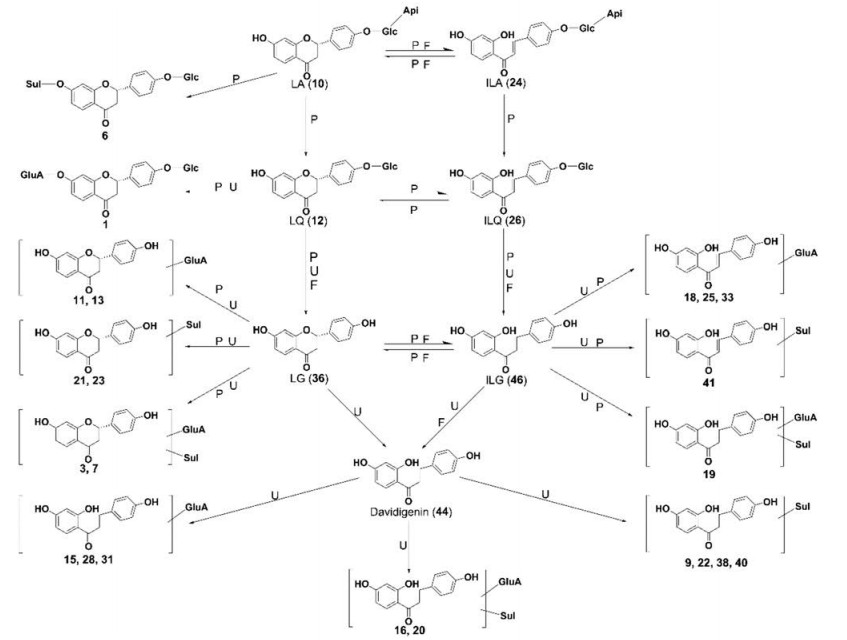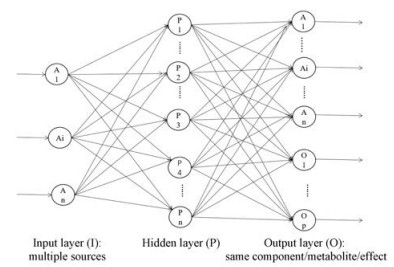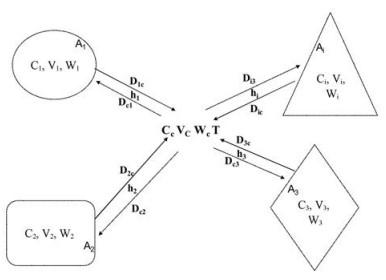﻿ 中药复杂系统的“多源归一”理论假说
 药学学报2019, Vol. 54Issue (5): 801-807     DOI: 10.16438/j.0513-4870.2018-1008PDF

1. 昆明理工大学生命科学与技术学院, 云南 昆明 650500;
2. 昆明理工大学信息工程与自动化学院, 云南 昆明 650500

Hypothesis on "one output multi-source" of traditional Chinese medicine complex system
XIANG Cheng1, LI Yong2, LI Kun2
1. Faculty of Life Science and Technology, Kunming University of Science and Technology, Kunming 650500, China;
2. Faculty of Information Engineering and Automation, Kunming University of Science and Technology, Kunming 650500, China
Abstract: Based on special scientific facts demonstrated in traditional Chinese medicine (TCM) complex system, this paper proposes a hypothesis of "one output multi-source", discussing the concept, features, structures and the scope. The law of interaction between the integrity and multiple components of TCM complex system was examined. Feasibility, technical methods and evidence supporting the hypothesis have been presented here. We present a basic model of the hypothesis, i.e. artificial neural network (ANN) model. This hypothesis promotes a deeper modern scientific understanding towards the TCM complex system and advancement in research of the material basis. TCM compatibility and quality control will serve as the theoretical foundation for guiding the research on drug combination including chemical, biological and herbal medicines.
Key words: traditional Chinese medicine     complex system     one output multi-source     artificial neural network     drug combination

1 “多源归一”假说的提出Figure 1 In vivo metabolic network of flavonoids in licorice 
2 中药复杂系统的“多源归一”假说的概念、特征和范围

3 检验“多源归一”假说的基本要求、基本模型、可能的技术方法和部分例证 3.1 论证“多源归一”假说的基本要求

“多源归一”假说的研究对象是中药复杂系统中一类特殊的相互作用, 该假说的证明应遵循科学假说检验的基本规则。按照系统科学理论, 若要检验该假说是否正确, 则需要先掌握单体化合物的特点, 再说明有多源归一现象的中药复杂系统的整体性、各个要素的相关性、系统内结构的层次性和有序性, 以及系统的动态变化规律

3.2 “多源归一”假说的基本模型Figure 2 Artificial neural network model of the hypothesis of one output multi-source for traditional Chinese medicine complex system

ANN是一种多层前馈网络, 是一种人工智能算法, 采用典型的、有指导学习的方式来进行预测和分类问题的处理。一个训练好的ANN, 理论上能够逼近任何自变量的网络输入和应变量的网络输出之间的函数关系。该模型在应用中可以识别变量间任意复杂的线性或非线性关系, 尤其是用现有的数学方法无法达到目的时, 采用神经网络模型往往能收到很好的效果。

3.3 不同时相的中药复杂系统“多源归一”假说的部分检验 3.3.1 药剂相的多源归一模型和检验Figure 3 Schematic diagram of multi-source normalization of the Chinese medicine compound. The compound contains herbs A1, A2, A3, …, Ai (indicated by different shape boxes), all of which contain the same component W, Ci is the concentration of the component W contained in the medicinal material i, and Vi is the internal volume of the medicinal material. During the boiling process, W is released to the central compartment Wc, and T is the boiling temperature

 $C\left( t \right) = \gamma \left( {{{\rm{e}}^{ - \alpha t}} - {{\rm{e}}^{ - \beta t}}} \right)$

 $\left\{ {\begin{array}{*{20}{c}} {{\rm{C}}\left( t \right) = \mathop \smallint \limits_0^t \alpha {C_{{\rm{in}}}}\left( t \right){\rm{dt}} - \mathop \smallint \limits_0^t \beta C\left( t \right){\rm{dt}}}\\ {{C_{{\rm{in}}}}\left( t \right) = {\rm{F}}{C_0} - \mathop \smallint \limits_0^{\rm{t}} \alpha {C_{{\rm{in}}}}\left( t \right){\rm{dt}}} \end{array}} \right.$

3.3.2 药效相的多源归一模型和检验

 $\frac{{fa}}{{fu}} = {\left[ {\frac{D}{{{D_m}}}} \right]^m}$

 ${\rm{CI}} = \frac{{\left( D \right)1}}{{\left( {{D_x}} \right)1}} + \frac{{\left( D \right)2}}{{\left( {{D_x}} \right)2}}$

 $\text{CI}=\sum\limits_{i=1}^{n}{{\frac{\left( D \right)i}{\left( {{D}_{x}} \right)i}}}$

3.3.3 药动相的多源归一模型和检验

 $\left\{ {\begin{array}{*{20}{c}} {{C_{M12}}\left( t \right) = \mathop \smallint \limits_0^t \alpha {C_{{\rm{in}}}}\left( t \right){\rm{dt}} - \mathop \smallint \limits_0^{\rm{t}} \beta C\left( t \right){\rm{dt}}}\\ {{C_{{\rm{M}}12{\rm{in}}}}\left( t \right) = {\rm{F}}{C_0} - \mathop \smallint \limits_0^t \alpha {C_{{\rm{in}}}}\left( t \right){\rm{dt}}} \end{array}} \right.$

 ${C_{M12}}\left( t \right){\rm{ }} = \gamma ({{\rm e}^{ - \alpha t}} - {{\rm e}^{ - \beta t}})$

 $C_{\rm{a}}^{\rm{'}} = \frac{1}{{{\rm{CI}}}}\left( {{C_{\rm{a}}} + k{C_{\rm{b}}}} \right)$

 $\left\{ {\begin{array}{*{20}{c}} {C_{\rm{a}}^{\rm{'}} = \frac{1}{{{\rm{CI}}}}\left( {{C_{\rm{a}}} + k{C_{\rm{b}}}} \right)}\\ {{f_{{\rm{a 预测}}}} = \frac{1}{{1 + {{\left( {\frac{{C_{\rm{a}}^{\rm{'}}}}{{{D_{m\left( {\rm{a}} \right)}}}}} \right)}^m}}}} \end{array}} \right.$

4 “多源归一”假说的意义和应用

  Miao MS, Ma RJ, Wei RR, et al. Research technique and consideration the proportion of the TCM components[J]. J Henan Univ (Med Sci) (河南大学学报(医学版)), 2011, 30: 1–5.  Ye ZG. Multi-ingredient traditional Chinese medicines preparations and their effective fractions[J]. Chin J New Drugs (中国新药杂志), 2011, 20: 1487–1489.  Liang XM, Xu Q, Xue XY, et al. Systematic research on the multi-component Chinese medicine[J]. World Sci Technol-Modern Tradit Chin Med Mater Med (世界科学技术-中医药现代化), 2006, 8: 1–7.  Fu JH, Fu Y, Liu JX. Conception of TCD component hypothesis[J]. Chin J Inform TCM (中国中医药信息杂志), 2006, 13: 52–54.  Kong L, Li BC, Xiang C. Research progress on effective compounds group of Chinese materia medica[J]. Chin Tradit Herb Drugs (中草药), 2015, 46: 3289–3296.  Wang YL. Systems Engineering (系统工程)[M]. 4th ed. Beijing: Mechanical Industry Press, 2008.  Hao HP, Zheng CN, Wang GJ. Thoughts and experimental exploration on pharmacokinetic study of herbal medicines with multiple-components and targets[J]. Acta Pharm Sin (药学学报), 2009, 44: 270–275.  He FY, Luo WJ, Deng KW, et al. A survey on status and research method of pharmacokinetics of formula Chinese materia medica[J]. Chin Tradit Herb Drugs (中草药), 2005, 36: 1582–1586.  Xu F, Yang DH, Shang MY, et al. Effective forms, additive effect, and toxicities scattering effect of pharmacodynamic substances of TCMs some reflections evoked by the study on the metabolic disposition of traditional chinese medicines (TCM)[J]. World Sci Technol-Modern Tradit Chin Med Mater Med (世界科学技术-中医药现代化), 2014, 16: 688–703.  He FY, Deng KW, Liu WL, et al. Reactive essence between Chinese materia medica formula and human body:multiple genetic chromatodynamokinetics with 'Co-network Compatibility and Rainbow Potential'[J]. Chin J Exp Tradit Med Formu (中国实验方剂学杂志), 2011, 17: 240–247.  Li QQ. Zuo Jinfang Multi-alkali In Vivo Network Metabolism " Rain Potential" Research (左金方多生物碱体内网络代谢"虹势性"研究)[D]. Changsha: Hunan University of Chinese Medicine, 2009. http://cdmd.cnki.com.cn/Article/CDMD-10541-2009243292.htm  Kang SY, Zhang JQ, Zhang AJ, et al. Based on urine metabolomics to study effect of Liuwei Dihuang Wan on growth and development of rats[J]. Acta Pharm Sin (药学学报), 2018, 53: 1713–1720.  Xiang Z, Cai XJ, Zeng S. Thoughts and exploration on pharmacokinetic study of herbal medicines based on metabonomics and complex network[J]. Acta Pharm Sin (药学学报), 2012, 47: 558–564.  Pang XC, Wang Z, Fang JS, et al. Network pharmacology study of effective constituents of traditional Chinese medicine for Alzheimer's disease treatment[J]. Acta Pharm Sin (药学学报), 2016, 51: 725–731.  Xiang C, Qiao X, Wang Q, et al. From single compounds to herbal extract:a strategy to systematically characterize the metabolites of licorice in rats[J]. Drug Metabol Disposit, 2011, 39: 1597–1608. DOI:10.1124/dmd.111.038695  Qiao X, Ye M, Xiang C, et al. Analytical strategy to reveal the in vivo process of multi-component herbal medicine:a pharmacokinetic study of licorice using liquid chromatography coupled with triple quadrupole mass spectrometry[J]. J Chromatogr A, 2012, 1258: 84–93. DOI:10.1016/j.chroma.2012.08.041  Fu SP, Wang LX, Zhang F, et al. Determination of ligustrazin and ferulic acid in Si-wu-tang and its related preparations by capillary zone electrophoresis[J]. Chin J Chromatogr (色谱), 2003, 21: 371–374.  Spiro M, Page CM. The kinetics and mechanism of caffeine infusion from coffee:hydrodynamic aspects[J]. J Sci Food Agr, 1984, 35: 925–930. DOI:10.1002/(ISSN)1097-0010  Spiro M, Jaganyi D. Kinetics and equilibria of tea infusion, Part 15. Transport of caffeine across a teabag membrane in a modified rotating diffusion cell[J]. Food Chem, 2000, 69: 119–124. DOI:10.1016/S0308-8146(99)00251-4  Spiro M, Chong YY. The kinetics and mechanism of caffeine infusion from coffee:the temperature variation of the hindrance factor[J]. J Sci Food Agr, 1997, 74: 416–420. DOI:10.1002/(ISSN)1097-0010  Chu MQ, Liu GJ. Kinetic model for extraction process of Chinese traditional medicine[J]. Acta Pharm Sin (药学学报), 2002, 37: 559–562.  He FY, Deng KW, Luo JY, et al. Fundamentally study on mathematical kinetic model of component extraction from FTCM[J]. China J Chin Mater Med (中国中药杂志), 2007, 32: 490–495.  Wang BC, Su H, Yang X, et al. Kinetic models for extraction process of natural products[J]. Chin Tradit Patent Med (中成药), 2012, 34: 2309–2312.  He FY, Deng KW, Wu DZ, et al. Extraction kineticai deviations of berberine alkaloids in Rhizoma Coptidis and Zuojin Formulae[J]. Chin Tradit Patent Med (中成药), 2009, 31: 1354–1359.  Yuan ST, Du HY, Xia K. The effect of Chinese herbal compound decoction on the dissolution effect[J]. Chin J Inform TCM (中国中医药信息杂志), 1999, 6: 29–32.  Tang ZS, Guo LW. Establishment of scientific principles on evaluation system for compound Chinese materia medica extraction and separation[J]. Chin Tradit Herb Drugs (中草药), 2010, 41: 841–845.  Su H, Wang BC, Liu WQ, et al. Kinetic mathematical models for extraction process of natural medicines[J]. Chin Tradit Herb Drugs (中草药), 2011, 42: 384–391.  Luo GA, Wang YM, Liang QL, et al. Chinese Medicine Systems Biology (中医药系统生物学)[M]. Beijing: Science Press, 2011.  Chou TC. Theoretical basis, experimental design, and computerized simulation of synergism and antagonism in drug combination studies[J]. Pharmacol Rev, 2006, 58: 621–681. DOI:10.1124/pr.58.3.10  Chou TC, Martin N. CompuSyn for drug combinations PC software and user's guide: a computer program for quantitation of synergism and antagonism in drug combinations, andthe determination of IC50 and ED50 and LD50 values[J/OL]. Paramus: ComboSyn, 2005.  Long F, Yang H, Xu YM, et al. A strategy for the identification of combinatorial bioactive compounds contributing to the holistic effect of herbal medicines[J]. Sci Rep, 2015, 5: 12361. DOI:10.1038/srep12361  Lam W, Jiang ZL, Guan FL, et al. PHY906 (KD018), an adjuvant based on a 1800-year-old Chinese medicine, enhanced the anti-tumor activity of sorafenib by changing the tumor microenvironment[J]. Sci Rep, 2015, 5: 9384. DOI:10.1038/srep09384  Gong P, Cui N, Wu L, et al. Chemicalome and metabolome matching approach to elucidating biological metabolic networks of complex mixtures[J]. Anal Chem, 2012, 84: 2995–3002. DOI:10.1021/ac3002353  Chen HX, Cui FX, Li H, et al. Metabolic changes during the pu-erh tea pile-fermentation revealed by a liquid chromatography tandem mass-spectrometry-based metabolomics approach[J]. J Food Sci, 2013, 78: C1665–C1672. DOI:10.1111/1750-3841.12288  Yan R, Yang Y, Chen YJ. Pharmacokinetics of Chinese medicines:strategies and perspectives[J]. Chin Med, 2018, 13: 24. DOI:10.1186/s13020-018-0183-z  Li C. Multi-compound pharmacokinetic research on Chinese herbal medicines:approach and methodology[J]. China J Chin Mater Med (中国中药杂志), 2017, 42: 607–617.